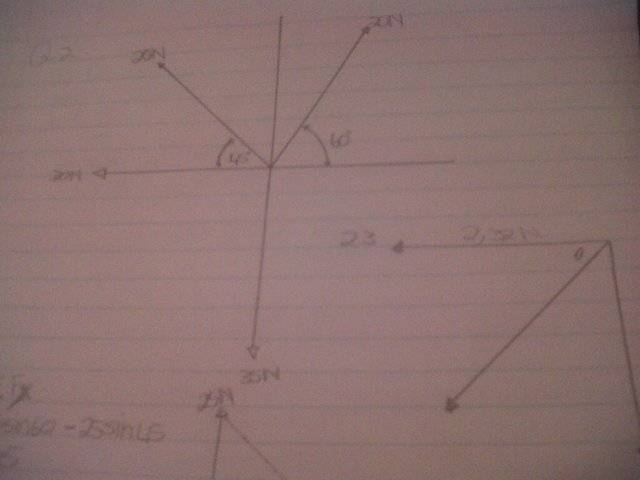# Four forces acting on a point

Sl1ver

## Homework Statement

I have done the following and would just like to know if it looks correct and any advice would be appreciated

Four forces acting on a point
30N west
35N south
20N west 45deg north
25N east 60deg north

1. The sum of the vertical components and state the direction
2. The sum of the horizontal components and state the direction
3. The magnitude of the resultant
4. The direction of the resultant
5. The magnitude and direction of the equilibrant

## The Attempt at a Solution

Please note that I haven't done physics in 5 years and busy getting back into it

here is the diagram I came up with1. 20sin60 - 25sin45 - 35N
= 17,321N - 17,678N - 35N
= -35,357N
= 35,357N South

2. 20cos60 + 25cos45 - 30N
= 10N + 17,678N - 30N
= - 2,322N
= 2,322N West

3. R² = 35,357² + 2,322²
R = √35,357 + √2,322
= √37,679
= 6,138N

4 and 5 I need help in the right direction.

any help would be appreciated.

Homework Helper

You need to pick two directions to be "positive" ... I'd pick "North" and "West" but some people will pick "East" to be positive since that looks like an x-axis.

A 45deg triangle has sides 1-1-√2
A 60-30 triangle has sides 1-2-√3

so. sin(45)=cos(45)=1/√2
so. sin(60)= √3/2, cos(60)=1/2

For 4 and 5:
Once you have the total of the N-S and E-W components (and their directions) - draw them in on your graph.

From there you can draw in the resultant.
The resultant arrow will tell you how to get the direction.
To see what is expected - look at how the question describes the magnitude and direction of vectors that are not exactly on a N-S or E-W axis.

Sl1ver
Thank you for the feedback. I appreciate the advice!

Is the graph I drew correct?

can you maybe just explain to me what you mean with
"A 45deg triangle has sides 1-1-√2
A 60-30 triangle has sides 1-2-√3

so. sin(45)=cos(45)=1/√2
so. sin(60)= √3/2, cos(60)=1/2"

Homework Helper
The graph you drew appears to be what was intended.
You have calculated the components of the resultant already - draw them on a similar graph. You are basically reversing the procedure you just used.

All trigonometry is based on right-angled triangles.
The angles used in the problem relate to a set of "special triangles" that have well-known and easily remembered properties. I brought it up because I wasn't sure you'd noticed.

----------------------
45-45-90 is what you get cutting a square in half corner-to-corner.
The ratio of it's sides are 1:1:√2 - the longest side is always the hypotenuse.

By definition: if the length of the hypotenuse is 1, then the length of the opposite side is the sine of the angle; if the adjacent side is 1, then the opposite side is the tangent of the angle and the hypotenuse is the secant. That is what those trig functions mean.

So:
tan(45)=1, and sec(45)=√2, can be read right off ...
sin(45)=1/√2 is obtained from similar triangles.
... no need for a calculator ;)

If you put two squares side-by-side to make an oblong, and cut the oblong corner-to-corner, you get a 30-60-90 triangle.
The ratio of the sides are 1-2-√3

Thus: tan(60)=√3 ... etc.

Whenever you see the angles 30, 45, or 60, you should think of these triangles. Also if you have a triangle where the two short sides are the same, or where one short side is half the hypotenuse.

Also learn 3-4-5 and 5-12-13.
3-4-5 triangles come up a lot!

http://www.onlinemathlearning.com/special-right-triangles.html